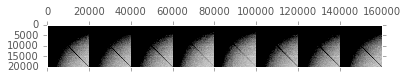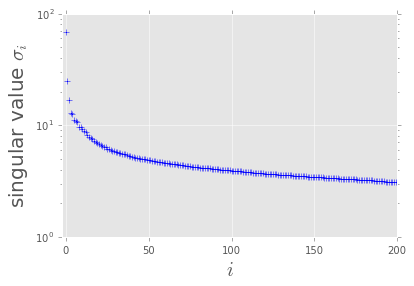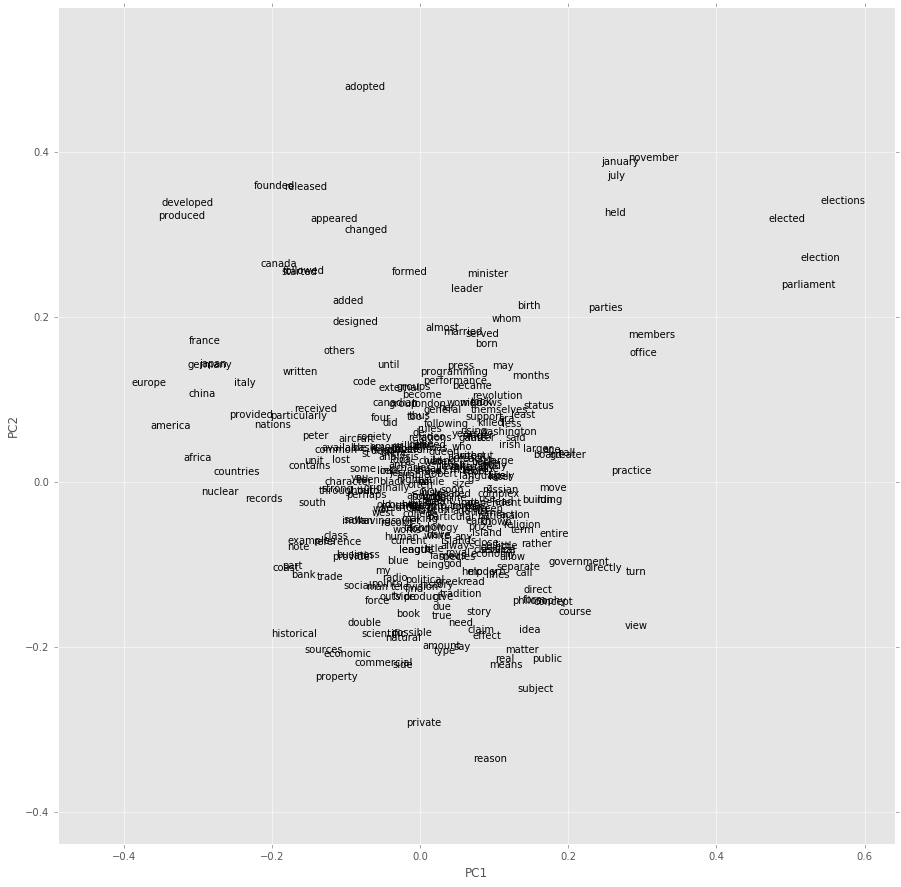# Implementation of Eigenwords (One-Step CCA)¶

## Requirements¶

$\newcommand{\mt}{\boldsymbol{\mathrm{#1}}}$

In :
import gc
import datetime as dt
from collections import Counter

import numpy as np
from scipy import sparse
import pandas as pd

from sklearn.decomposition import PCA
from sklearn.utils.extmath import randomized_svd
from sklearn.preprocessing import normalize

import matplotlib.pyplot as plt
import matplotlib
matplotlib.style.use('ggplot')
%matplotlib inline

datetime_start = dt.datetime.today()

In :
path_corpus = './text8'

# tuning parameters
max_size_vocabulary = 20000
dim_embedding = 200
size_window = 4

In :
# Load a text file
with open(path_corpus, 'r') as f:
txt = txt[1:].split(' ')

# Retrieve vocabulary
vocabulary = ['<OOV>']
counter = Counter(txt)

for word, n in counter.most_common():
if len(vocabulary) >= max_size_vocabulary:
break

vocabulary.append(word)

print('# of word types : {0}'.format(len(counter.items())))
print('Size of vocabulary : {0}'.format(len(vocabulary)))
print('\nTop 100 frequentry-used words:\n  ' + ' '.join(vocabulary[0:100]))

# of word types : 253854
Size of vocabulary : 20000

Top 100 frequentry-used words:
<OOV> the of and one in a to zero nine two is as eight for s five three was by that four six seven with on are it from or his an be this which at he also not have were has but other their its first they some had all more most can been such many who new used there after when into american time these only see may than world i b would d no however between about over years states people war during united known if called use th system often state so history will up while

In :
size_vocabulary = len(vocabulary)
vocab_to_id = dict(zip(vocabulary, range(size_vocabulary)))
id_tokens = np.array([vocab_to_id[t] if t in vocab_to_id else 0 for t in txt], dtype=np.int64)

In :
%%cython

import numpy as np
cimport numpy as np
from scipy import sparse

def construct_matrices(long[:] id_tokens, int size_vocabulary, int size_window):
cdef:
long long i_token, n_pushed = 0, n_tokens = len(id_tokens)
long long id_word, id_context, i_offset, offset
np.ndarray[np.int64_t, ndim=1] offsets = np.array(
[i for i in range(-size_window, size_window + 1) if i != 0], dtype=int)
np.ndarray[np.int64_t, ndim=1] VTV_diag = np.zeros(size_vocabulary, dtype=int)
np.ndarray[np.int64_t, ndim=1] CTC_diag = np.zeros(2*size_window*size_vocabulary, dtype=int)
np.ndarray[np.int64_t, ndim=1] VTC_row_index = np.empty(2*size_window*n_tokens, dtype=int) # undesirable
np.ndarray[np.int64_t, ndim=1] VTC_col_index = np.empty(2*size_window*n_tokens, dtype=int) # undesirable

for i_token in range(n_tokens):
id_word = id_tokens[i_token]
VTV_diag[id_word] += 1

for i_offset in range(2*size_window):
offset = offsets[i_offset]
if not (0 <= i_token + offset < n_tokens): continue
id_context = id_tokens[i_token + offset] + i_offset * size_vocabulary

CTC_diag[id_context] += 1
VTC_row_index[n_pushed] = id_word
VTC_col_index[n_pushed] = id_context
n_pushed += 1

VTC_values =  * n_pushed
VTC = sparse.csc_matrix(
(VTC_values, (VTC_row_index[range(n_pushed)], VTC_col_index[range(n_pushed)])),
shape=(size_vocabulary, 2*size_window*size_vocabulary))

return (VTV_diag, CTC_diag, VTC)

In :
VTV_diag, CTC_diag, VTC = construct_matrices(id_tokens, size_vocabulary, size_window)
gc.collect()

Out:
50
\begin{align} \mt{\mathcal{C}}_{VV} = \sqrt{\mt{V}^\top \mt{V}}, \quad \mt{\mathcal{C}}_{VC} = \sqrt{\mt{V}^\top \mt{C}}, \quad [\mt{\mathcal{C}}_{CC}]_{i, j} = \delta_{i, j} \cdot \left[\sqrt{\mt{C}^\top \mt{C}}\right]_{i, j} \end{align}

The optimal solution of One-Step CCA can be obtained via SVD of $\mt{A} = \mt{\mathcal{C}}_{VV}^{-1/2} \mt{\mathcal{C}}_{VC} \mt{\mathcal{C}}_{CC}^{-1/2}$. ($\sqrt{\cdot}$ indicates element-wise sqrt, and $\delta$ is Kronecker delta.)

In :
VTV_diag_h = VTV_diag.astype('double')**(-1/4)
CTC_diag_h = CTC_diag.astype('double')**(-1/4)
A = sparse.diags(VTV_diag_h) @ VTC.power(1/2) @ sparse.diags(CTC_diag_h)

In :
A

Out:
<20000x160000 sparse matrix of type '<class 'numpy.float64'>'
with 31160604 stored elements in Compressed Sparse Row format>
In :
plt.spy(A, markersize=0.005, alpha=0.3)

Out:
<matplotlib.lines.Line2D at 0x7f35f9cd4d30>In :
U, S_diag, V = randomized_svd(A, n_components=dim_embedding, n_iter=3)

In :
print('Elapsed time :', dt.datetime.today() - datetime_start)

Elapsed time : 0:02:33.234541

In :
plt.plot(S_diag, 'b+')
plt.xlim(-2, )
plt.yscale('log')
plt.xlabel('$i$', fontsize=20)
plt.ylabel('singular value $\sigma_i$', fontsize=20)

Out:
<matplotlib.text.Text at 0x7f35f97a0240>In :
vectors = pd.DataFrame(normalize(sparse.diags(VTV_diag_h) @ U), index=vocabulary)

In :
query = 'godzilla'

similarities = pd.Series(vectors.values @ vectors.loc[query, :].values, index=vocabulary)

Out:
godzilla          1.000000
batman            0.662502
toho              0.647189
akira             0.629874
blaxploitation    0.614074
animated          0.608280
movie             0.607745
monster           0.604997
anime             0.601940
kurosawa          0.600349
dtype: float64
In :
# biplot
margin_plot = 0.1

vectors_plot = vectors.iloc[np.arange(0, 1000, 3), :]
pca = PCA(n_components=2)
X = pca.fit_transform(vectors_plot)

fig = plt.figure(figsize=(15, 15))

for i in range(X.shape):
ax.text(X[i, 0], X[i, 1], vectors_plot.index[i])

_ = ax.axis([min(X[:, 0]) - margin_plot, max(X[:, 0]) + margin_plot,
min(X[:, 1]) - margin_plot, max(X[:, 1]) + margin_plot])

ax.set_xlabel('PC1')
ax.set_ylabel('PC2')

Out:
<matplotlib.text.Text at 0x7f35a9f8e438>## Reference¶

• Paramveer S. Dhillon, Dean P. Foster, and Lyle H. Ungar. 2015. Eigenwords: Spectral word embeddings. Journal of Machine Learning Research, 16:3035–3078.